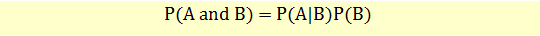# Joint Probability of Two Events

Joint probability refers to the multiplication rule of probability. This is the probability that both the events will occur.Example

The probability that the price of oil will rise, P(B) = 0.5

The probability that the bus fare will increase if oil price rises, P(A|B) = 0.4

The probability that both oil prices and bus fares will rise, P(AB) = 0.4*0.5 = 0.2

This may look complex but the logic is actually quite straight forward. There is a 50% chance that oil price will rise and if it rises there is a 40% chance that the bus fair will also rise. So, the joint probability of both oil price rise and bus fare rise is 50% of 40%, i.e., 0.5*0.4 = 0.20 or 20%.

Get our Data Science for Finance Bundle for just $29$51.